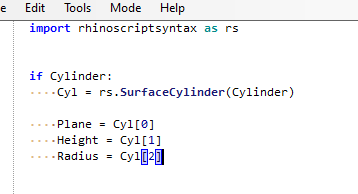# Finding axis of points on a cylinder

I have a collection of points which I know lie exactly on a cylinder, but I don’t know the cylinder’s orientation, radius, or nationality. Using `Fit Line`, which i assume uses Least Squares, I get an axis but in comparing the result to an actual cylinder, there is a difference, though small. `Fit Line` is meant for a scatter of points, whereas I already know they are on a cylinder, so there is some regularity. It seems with that information I should be able to do even better. Then again, maybe not…

determine axis.gh (12.9 KB)

I’m guessing the point cloud would come from a scan?

Here is a closer approximation using Kangaroo.

determine axis_V2.gh (19.4 KB)

Yeah…I feel like there is a good mathematical solution there somewhere…but I just cant think of it right now.

7 Likes

Very nice Adam! It works well with even far fewer points than in the example. Looks like insisting the radii are equal makes the difference. My actual points are generated iteratively and, strange as it sounds, all I do know is they lie around a cylinder. I’m working on an analytic expression for the radius, but until (unless) I do, this will suit me quite well. Ultimately, I want to orient the points along the z axis, which is why I needed the cylinder’s axis.

What if you construct a minimum bbox out of the points and then take the area centroids of the two smallest faces?

Uh, no. You could have tried it before posting…Good point, then as a base of the Bbox take one of the faces of the cylinder.

If the faces of the cylinder were known, there wouldn’t be a problem at all.

My bad there, I posted this from my phone.

It actually makes sense not to align the axis properly. The fewer the points the bigger the deviation.

1 Like

And you’re kinda right. Feature detection of cylinders in point clouds is a topic with a plethora of papers · written · about it.
But beware: The ‘solution’ is really math intense – your’s better I think(and also easier to grok).

1 Like

This is another “simple” way to achieve something, with galapagos… just for reference.

determine axis_galapagos.gh (17.7 KB)

Adam’s solution is way better in every mean.

3 Likes

Also very clever Riccardo! The axis angle and radius are almost exact.

@akilli I also had to solve this cylinder fit problem.

I believe the whole problem could be simplified to this code, where Pts are points and n are iterations.

`````` private void RunScript(List<Point3d> Pts, int n, ref object LFit, ref object LfitCP)
{

Line lfit = Line.Unset;
Line.TryFitLineToPoints(Pts, out lfit);

Point3d[] lfitCP = new Point3d[Pts.Count];

for(int k = 0; k < n; k++){
//1.0 Fit Points to aline
double averageDist = 0;
for(int i = 0; i < Pts.Count; i++){
Point3d plfit = lfit.ClosestPoint(Pts[i], false);
lfitCP[i] = plfit;
averageDist += plfit.DistanceTo(Pts[i]);
}
averageDist /= Pts.Count;

//2.0 Move line point by equal dist
for(int i = 0; i < Pts.Count; i++){
Vector3d v = lfitCP[i] - Pts[i];
v.Unitize();
v *= averageDist;
lfitCP[i] = Pts[i] + v;
}

Line.TryFitLineToPoints(lfitCP, out lfit);
}

//repeat

LFit = lfit;
LfitCP = lfitCP;
}
``````

here’s one non-loopy approachfind_axis_of_cylinder.gh (25.9 KB)

If you are sure that the shape is cylinder you can use GhPython to get Plane, Height and Radius

determine cylinder axis.gh (12.0 KB)1 Like

cheater!1 LikeIf you have points only you can project them in XY, XZ,YZ planes and use the projected points to find the center line